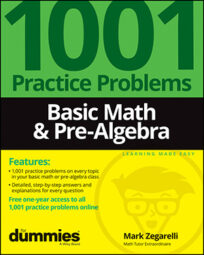##### Basic Math & Pre-AlgebraDecimals are commonly used for money, as well as for weights and measures, especially when using the metric system. As you practice basic math and pre-algebra problems, you'll find that decimals are easier to work with than fractions.

• To change a decimal to a fraction, put the decimal in the numerator of a fraction with a denominator of 1. Then, continue to multiply both the numerator and denominator by 10 until the numerator is a whole number. If necessary, reduce the fraction.

• To change a fraction to a decimal, divide the numerator by the denominator until the division either terminates or repeats.

• To change a repeating decimal to a fraction, put the repeating portion of the decimal (without the decimal point) into the numerator of a fraction. Use as a denominator a number composed only of 9s with the same number of digits as the numerator. If necessary, reduce the fraction.

• To add or subtract decimals, line up the decimal points.

• To multiply decimals, begin by multiplying without worrying about the decimal points. When you're done, count the number of digits to the right of the decimal point in each factor and add the result. Place the decimal point in your answer so that your answer has the same number of digits after the decimal point.

• To divide decimals, turn the divisor (the number you're dividing by) into a whole number by moving the decimal point all the way to the right. At the same time, move the decimal point in the dividend (the number you're dividing) the same number of places to the right. Then place a decimal point in the quotient (the answer) directly above where the decimal point now appears in the dividend.

• When dividing decimals, continue until the answer either terminates or repeats.